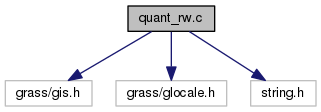GRASS Programmer's Manual  6.5.svn(2014)-r66266
quant_rw.c File Reference
`#include <grass/gis.h>`
`#include <grass/glocale.h>`
`#include <string.h>`
Include dependency graph for quant_rw.c:Go to the source code of this file.

## Functions

int G_truncate_fp_map (const char *name, const char *mapset)

int G_round_fp_map (const char *name, const char *mapset)

int G_quantize_fp_map (const char *name, const char *mapset, CELL min, CELL max)
Writes the `f_quant` file for the raster map name with one rule. The rule is generated using the floating-point range in `f_range` producing the integer range [cmin,cmax]. More...

int G_quantize_fp_map_range (const char *name, const char *mapset, DCELL d_min, DCELL d_max, CELL min, CELL max)
Writes the `f_quant` file for the raster map name with one rule. The rule is generated using the floating-point range [dmin,dmax] and the integer range [min,max]. This routine differs from the one above in that the application controls the floating-point range. For example, r.slope.aspect will use this routine to quantize the slope map from [0.0, 90.0] to [0, 90] even if the range of slopes is not 0-90. The aspect map would be quantized from [0.0, 360.0] to [0, 360]. More...

int G_write_quant (const char *name, const char *mapset, const struct Quant *quant)
Writes the `f_quant` file for the raster map name from q. if mapset==G_mapset() i.e. the map is in current mapset, then the original quant file in cell_misc/map/f_quant is written. Otherwise q is written into quant2/mapset/name (much like colr2 element). This results in map being read using quant rules stored in q from G_mapset(). See G_read_quant() for detailes. More...

int G_read_quant (const char *name, const char *mapset, struct Quant *quant)
reads quantization rules for `"name"` in `"mapset"` and stores them in the quantization structure `"quant"`. If the map is in another mapset, first checks for quant2 table for this map in current mapset. Return codes: -2 if raster map is of type integer -1 if (! G__name_is_fully_qualified ()) 0 if quantization file does not exist, or the file is empty or has wrong format. 1 if non-empty quantization file exists. More...

## Function Documentation

 int G_quantize_fp_map ( const char * name, const char * mapset, CELL min, CELL max )

Writes the `f_quant` file for the raster map name with one rule. The rule is generated using the floating-point range in `f_range` producing the integer range [cmin,cmax].

Parameters
 name cmin cmax
Returns
int

Definition at line 113 of file quant_rw.c.

 int G_quantize_fp_map_range ( const char * name, const char * mapset, DCELL d_min, DCELL d_max, CELL min, CELL max )

Writes the `f_quant` file for the raster map name with one rule. The rule is generated using the floating-point range [dmin,dmax] and the integer range [min,max]. This routine differs from the one above in that the application controls the floating-point range. For example, r.slope.aspect will use this routine to quantize the slope map from [0.0, 90.0] to [0, 90] even if the range of slopes is not 0-90. The aspect map would be quantized from [0.0, 360.0] to [0, 360].

Parameters
 name dmin dmax cmin cmax
Returns
int

Definition at line 159 of file quant_rw.c.

References buf, G_quant_add_rule(), G_quant_init(), G_warning(), G_write_quant(), and sprintf().

Referenced by G_quantize_fp_map(), IL_output_2d(), and IL_resample_output_2d().

 int G_read_quant ( const char * name, const char * mapset, struct Quant * quant )

reads quantization rules for `"name"` in `"mapset"` and stores them in the quantization structure `"quant"`. If the map is in another mapset, first checks for quant2 table for this map in current mapset. Return codes: -2 if raster map is of type integer -1 if (! G__name_is_fully_qualified ()) 0 if quantization file does not exist, or the file is empty or has wrong format. 1 if non-empty quantization file exists.

Parameters
 name mapset q
Returns
int

Definition at line 245 of file quant_rw.c.

References G__quant_import(), and G_quant_init().

 int G_round_fp_map ( const char * name, const char * mapset )

Definition at line 81 of file quant_rw.c.

References buf, G_quant_init(), G_quant_round(), G_warning(), G_write_quant(), and sprintf().

 int G_truncate_fp_map ( const char * name, const char * mapset )

Definition at line 64 of file quant_rw.c.

References buf, G_quant_init(), G_quant_truncate(), G_warning(), G_write_quant(), and sprintf().

 int G_write_quant ( const char * name, const char * mapset, const struct Quant * quant )

Writes the `f_quant` file for the raster map name from q. if mapset==G_mapset() i.e. the map is in current mapset, then the original quant file in cell_misc/map/f_quant is written. Otherwise q is written into quant2/mapset/name (much like colr2 element). This results in map being read using quant rules stored in q from G_mapset(). See G_read_quant() for detailes.

Parameters
 name mapset q
Returns
int

Definition at line 198 of file quant_rw.c.

References buf, G__quant_export(), G_quant_get_limits(), G_raster_map_type(), G_warning(), and sprintf().

Referenced by G_quantize_fp_map_range(), G_round_fp_map(), and G_truncate_fp_map().